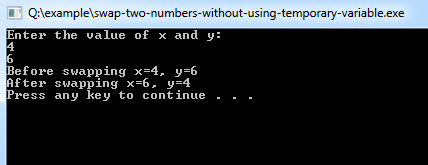## C++ Programming Examples Tutorial Index

This C++ program is used to demonstrates swapping two numbers by using a temporary variable.

Example:

``````#include <iostream>
using namespace std;

int main()
{
int x, y, temp;
cout << "Enter the value of x and y:" << endl; cin >> x >> y;
cout << "Before swapping x=" << x << ", y=" << y << endl;

/*Swapping logic */
temp = x;
x = y;
y = temp;
cout << "After swapping x=" << x << ", y=" << y << endl;
return 0;
}``````

Program Output:Explanation:

This program is showing the use of swapping of 2 variables using a temporary variable. So, first of all, you have to include the iostream header file using the "include" preceding by # which tells that hat the header file needs to be process before compilation, hence named preprocessor directive. Now, for removing naming conflict you can use namespace statement within a program.

Next you start writing the code and declare the main() with its return typeas'int'. Now, you have to declare three integer type variables name 'x', 'y',and'temp'. Then the cout<<""; statement is used which is used to print the message:
"Enter the value of x and y".

The cin>> statement then takes the values of X and Y from the user and using cout statement shows the value of x and y before swpping. The 'endl' is used to command the C++ compiler to end the line and move the cursor to the next line. Now the swapping logic is implemented where the 'temp' stores the value for 'x'. Then the value of 'y' is stored in 'x'. And finally the value of 'temp' which was first initialized by 'x' is now stored in 'y'. Here the 'temp' which is a temporary variable acts an a temporary container of the variable of 'x'.

Now the cout<<""; statement is again used to print the swapped values of 'x' and 'y' on to the screen. . The system("PAUSE") is used to pause the console of the system. And finally the return 0; statement is used to return an integer type value back to main().